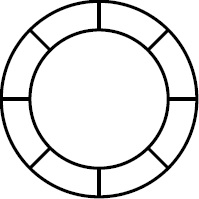# Blue clues

Probability Level 5

Consider the circular grid shown in the figure below. A red token is placed into the first outer spot clockwise from the top. A set of $k$ blue tokens for some $0 \leq k \leq 8$ are then placed into spots on the grid such that no two blue tokens are in the same spot, but a blue token may be on the same spot as the red token.Armin planned to flip ten fair coins and for each head that appeared, he would move the red token one position clockwise. Before flipping the coins, Armin (correctly) calculated that the probability of the red token ending in the same position as a blue token was $\frac{a}{b}$ where $a$ and $b$ are integers such that $a + b = 1400$. Armin was a bit lazy with his calculation so the fraction $\frac{a}{b}$ might not have been in lowest terms.

Determine the number of different sets of spots which may contain blue tokens.

Details and assumptions

$a$ can be 0.

Clarification: Since the red token is fixed, the sets of spots obtained by reflection or rotation are considered distinct.

×